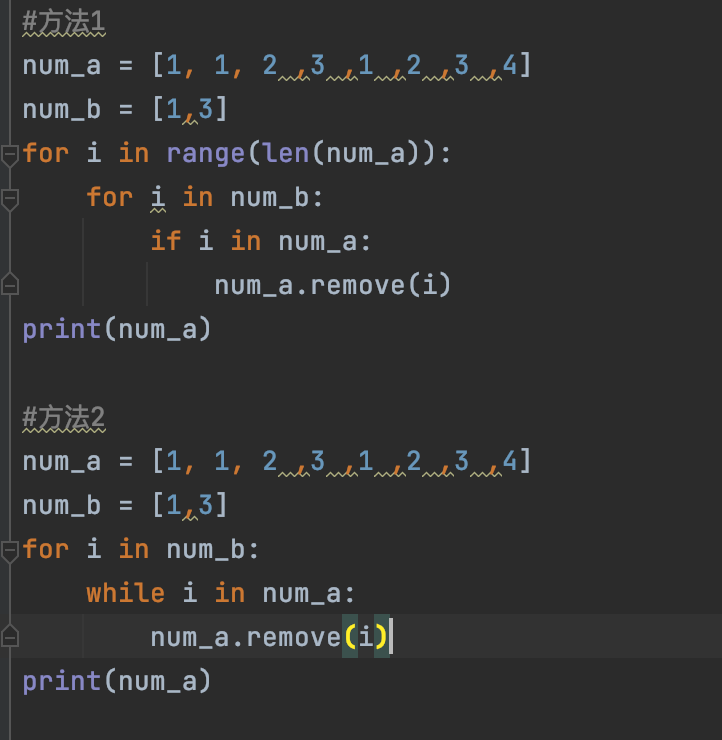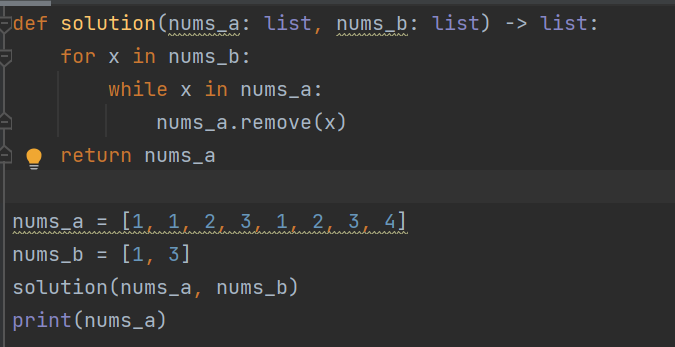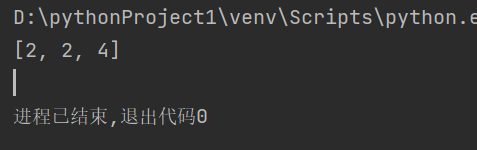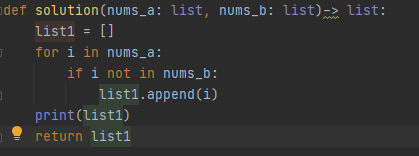# 【每日一题20220331】列表运算‍ 给定两个数字列表，请编写一个函数，从第一个列表nums_a中移除所有在第二个列表nums_b中出现的元素，返回剩下元素组成的列表。

【示例】

``````def solution(nums_a: list, nums_b: list)-> list:

assert solution([1, 1, 2 ,3 ,1 ,2 ,3 ,4], [1, 3]) == [2, 2, 4]
assert solution([8, 2, 7, 2, 3, 4, 6, 5, 4, 4, 1, 2 , 3], [2, 4, 3]) == [8, 7, 6, 5, 1]
assert solution([1, 1, 2 ,3 ,1 ,2 ,3 ,4, 4, 3 ,5, 6, 7, 2, 8], [1, 3, 4, 2]) == [5, 6 ,7 ,8]
``````
``````def solution(nums_a, nums_b):
return [item for item in nums_a if item not in nums_b]
``````
1 Like
``````def solution(nums_a: list, nums_b: list) -> list:
for x in nums_b:
while x in nums_a:
nums_a.remove(x)
return nums_a
``````
``````def solution(nums_a, nums_b):
map=Counter(nums_a)
for i in nums_b:
if i in map.keys():
map.pop(i)
return list(map.elements())

``````

def solution(nums_a: list, nums_b: list)-> list:

``````from collections import Counter
for x in nums_b:
for i in range(0,Counter(nums_a).get(x)):
nums_a.remove(x)
return nums_a

assert solution([1, 1, 2 ,3 ,1 ,2 ,3 ,4], [1, 3]) == [2, 2, 4]
assert solution([8, 2, 7, 2, 3, 4, 6, 5, 4, 4, 1, 2 , 3], [2, 4, 3]) == [8, 7, 6, 5, 1]
assert solution([1, 1, 2 ,3 ,1 ,2 ,3 ,4, 4, 3 ,5, 6, 7, 2, 8], [1, 3, 4, 2]) == [5, 6 ,7 ,8] `````````
``````    def solution(nums_a: list, nums_b: list) -> list:
return [i for i in nums_a if i not in nums_b]
``````
1 Like

def solution(nums_a:list, nums_b:list)->list:
lst = [i for i in nums_a if i not in nums_b]
return lst

1 Like``````
def solution(nums_a: list, nums_b: list)-> list:
for _ in range(len(nums_a)):
for i in nums_b:
for j in nums_a:
if i==j:
nums_a.remove(i)
return nums_a
``````
``````def solution(nums_a: list, nums_b: list)-> list:
return [i for i in nums_a if i not in nums_b]
``````
``````def solution(nums_a: list, nums_b: list)-> list:
return [var for var in nums_a if var not in nums_b]

assert solution([1, 1, 2 ,3 ,1 ,2 ,3 ,4], [1, 3]) == [2, 2, 4]
assert solution([8, 2, 7, 2, 3, 4, 6, 5, 4, 4, 1, 2 , 3], [2, 4, 3]) == [8, 7, 6, 5, 1]
assert solution([1, 1, 2 ,3 ,1 ,2 ,3 ,4, 4, 3 ,5, 6, 7, 2, 8], [1, 3, 4, 2]) == [5, 6 ,7 ,8]
``````

def solution(nums_a: list, nums_b: list)-> list:

``````for y in range(0,len(nums_b)):
for i in nums_a[:]:
if i == nums_b[y]:
nums_a.remove(i)
return nums_a``````
``````def solution(nums_a: list, nums_b: list) -> list:
return [x for x in nums_a if x not in nums_b]

assert solution([1, 1, 2, 3, 1, 2, 3, 4], [1, 3]) == [2, 2, 4]
assert solution([8, 2, 7, 2, 3, 4, 6, 5, 4, 4, 1, 2, 3], [2, 4, 3]) == [8, 7, 6, 5, 1]
assert solution([1, 1, 2, 3, 1, 2, 3, 4, 4, 3, 5, 6, 7, 2, 8], [1, 3, 4, 2]) == [5, 6, 7, 8]

``````
``````def solution(nums_a: list, nums_b: list) -> list:
new_list = []
for i in nums_a:
if i not in nums_b:
new_list.append(i)
return new_list
assert solution([1, 1, 2, 3, 1, 2, 3, 4], [1, 3]) == [2, 2, 4]
assert solution([8, 2, 7, 2, 3, 4, 6, 5, 4, 4, 1, 2, 3], [2, 4, 3]) == [8, 7, 6, 5, 1]
assert solution([1, 1, 2, 3, 1, 2, 3, 4, 4, 3, 5, 6, 7, 2, 8], [1, 3, 4, 2]) == [5, 6, 7, 8]
``````